Robotics 1
Cameras and Color
Quiz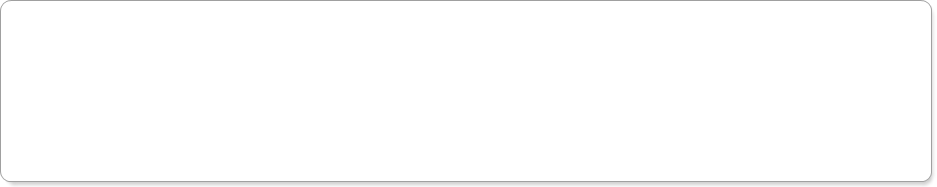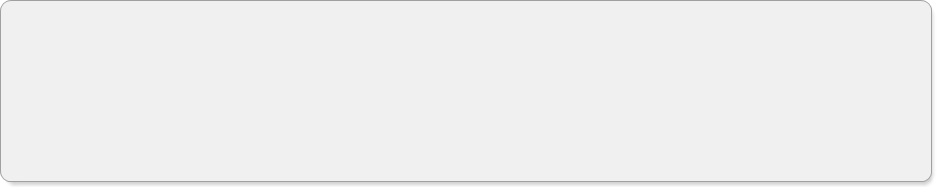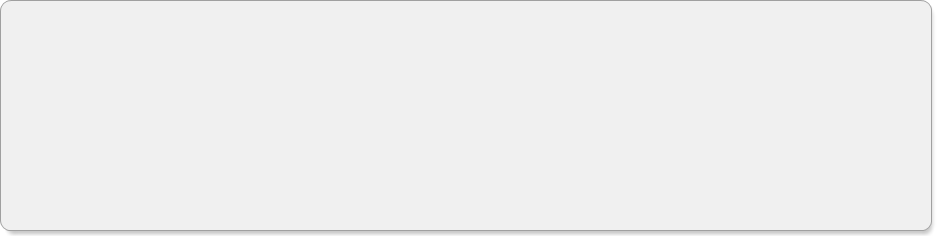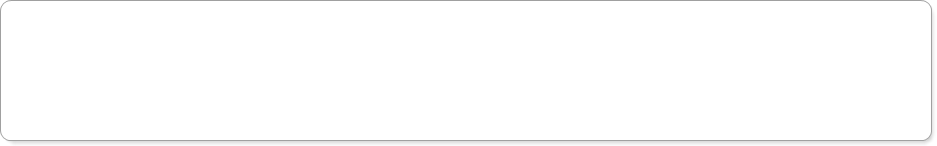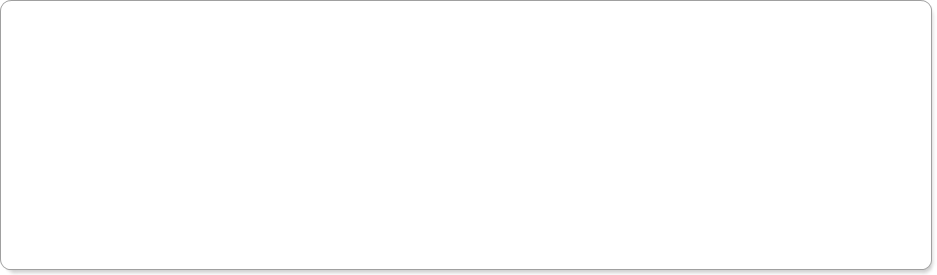Your ID Code:
Question 2:  What does the keyword 'break' do in Python?
Question 3:  For question 1, if each number is stored in an 8-bit value, how many bits of information does the image contain?  Don't include any commas, enter only numbers.
Question 4:  Suppose that we convert the image from questions 1 and 3 to an 8-bit grayscale image.  How many FEWER bits are in the grayscale image compared to the color image?  Don't include any commas, enter only numbers.
Question 5:  In the image matrix of a grayscale image, what does the value of each number represent?
Question 6:  In OpenCV, which 'layer' of an image matrix is the red layer?
Question 7:  If we look at only Layer 2 of the RGB image in OpenCV, what color object will show up the brightest? (remember that the first layer is 'Layer 0')
Question 8:  Which contains more bits of data (assuming both have an 8-bit depth)?
Question 9:  Suppose you have a 10-bit depth RGB image at a resolution of 1280x720, and you convert the image down to 8-bit depth (with the same color and resolution).  How many bits of information are lost?  Don't include any commas, enter only numbers.
Question 10:  In which 'layer' does the color white show up the brightest?  (This is a 'foreshadowing' question to set up the concepts we will be learning in the next section).
Undergraduate/Graduate Questions (both undergraduates AND graduates should answer these questions):
Question 11: In the RGB panel, slide the R slider all the way to the right, and slide the G and B sliders all the way to the left, so that you get a completely red color.  Then, look down at the HSV panel.  What are the HSV values that correspond to this red color?
Question 12:  Now, starting with the sliders the way they are at the end of Question 11, find the HSV panel (make sure NOT to use the HSL panel).  Slide the saturation slider left and right and watch what happens to the R, G, and B sliders.  What do you observe?
Question 13:  After finishing Question 12, leave the saturation slider in the right-most position.  Now, slide just the Value slider left and right and watch what happens to the R, G, and B sliders.  What do you observe?
Question 14:  Now let's do some explorations with YUV.  Find the panel called 'YPbPr (similar to YCbCr & YUV).  In this panel, the Pr slider is U, and the Pb slider is V.  Slide all three sliders all the way to the left.  When the U slider (Pr) is slid, which two colors (chromas) are you selecting between?
Question 15: Now, place all three YUV sliders back to the left, and slide only the V (Pb) slider.  Which two colors (chromas) are you selecting between?
Question 1: If you take a color image at a resolution of 640x480, how many numbers will be contained in the matrix that represents the image?  Don't include any commas, enter only numbers.
'break' makes the program end
'break' escapes an error in the program
'break' makes an if statement end
'break' exits the loop it is in
The pixel position
The color of the pixel
The pixel brightness
The size of the pixel
Layer 0
Layer 1
Layer 2
Layer 3
red objects
green objects
blue objects
An RGB image with resolution of 640x480
A grayscale image with resolution of 1920x1080
Graduate Questions (ONLY graduates need to answer these questions):
Layer 0
Layer 1
Layer 2
White is equally bright in all layers
Let's do some exploration of color spaces.  Open up a web browser and go to http://colorizer.org/.  This site easily allows you to convert between RGB, HSV, and YUV.  These questions will ask you to do five 'explorations' of the relationships between these three color spaces.
Hue is maximum, Saturation and Value are minimum
Hue is minimum, Saturation and Value are maximum
Saturation is maximum, Hue and Value are minimum
Saturation is minimum, Hue and Value are maximum
Only the Green slider moves
Only the Green and Blue sliders move
Only the Red slider moves
The Red, Green, and Blue sliders move together
Only the Green slider moves
Only the Green and Blue sliders move
Only the Red slider moves
The Red, Green, and Blue sliders move together
Blue and Green
Red and Green
Red and Blue
Blue and Yellow
Blue and Green
Blue and Yellow
Red and Blue
Red and Green